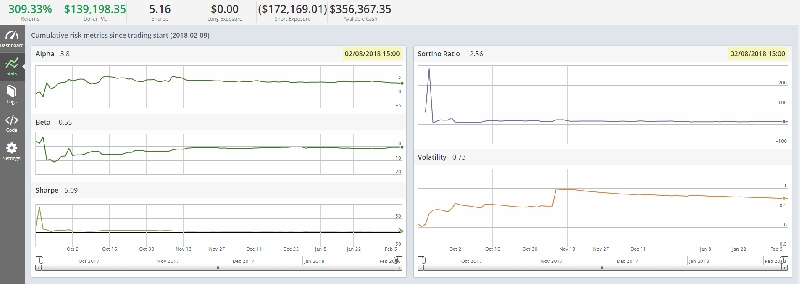My code shown below has been modified to mask the original values, I'll explain the functions of the mentioned code and ultimately I'd like to have this be ported to QuantConnect to be traded using Interactive BrokersThe strategy is a Sole Short Selling strategy, in the code just for the sake of proving a point a backtest was initiated on 09/08/2017 utilizing only market orders. This was important to determine the ability to #1 get filled and #2 see if the most unreasonable execution could yield a profit.

The strategy also relies on there being shares available for shorting at Interactive Brokers

Code Function Breakdown -

Call Open, High, Low, Close, Volume Data

Calculate last day's momentum - Unreasonable, however it has proven profitable

Compute corporate values (book,sales,free cash flow) morning star - sort worst to best

With corporate values determine quality gross profit, balance sheet totals - sort worst to best

Using pandas create a custom volatility factor generated using the last 3 trading day closes.

- Startup

Rank results based on momentum and utilize only those with a momentum below 0.7 pick the top 5

Universe filter - Average volume above N for liquidity

call the last price to be used in the universe filter

calculate average volume on as a 5 day sma

calculate RSI in a percentage

OH Range - 5 day HIGH SMA MINUS 5 DAY OPEN SMA

mean_range = OH Range divided by last price * 100

call value

call momentum

call volality

filter by vol_range is average volume > greater than a set value to ensure liquidity

filter by rsi_range = rsi percent greater than and less than set values

filter by OH_range_percent - mean range between two predefined values

define dollar volume = averagedollarvolume(5 day sma)

filter by high_volume = dollar_volume percentile between 20,90

define price = usequity.close.latest

filter by at most price

filter by at least price

initiate pipe screen to include

high_volume, at most price, at least price, volume range, rsi_range, OH range

utilize the S&P as a bench mark

for an orderly market when managing larger sums implement slippage

the strategy rebalances every day on market open

open orders cancelled at market close

positions are closed out once the momentum factor exceeds the universe filter

In exchange for your assistance you will learn a new strategy and will receive the original quantopian code which yielded the above results exceeding 300% in less than one year.

```from quantopian.algorithm import attach_pipeline, pipeline_output from quantopian.pipeline import Pipeline from quantopian.pipeline.data.builtin import USEquityPricing from quantopian.pipeline.factors import CustomFactor, AverageDollarVolume from quantopian.pipeline.factors import SimpleMovingAverage from quantopian.pipeline.factors import RSI from quantopian.pipeline.data import morningstar from zipline.finance.asset_restrictions import HistoricalRestrictions, Restriction, RESTRICTION_STATES as states from datetime import timedelta import pandas as pd import numpy as np class Value(CustomFactor): inputs = [morningstar.valuation_ratios.book_value_yield, morningstar.valuation_ratios.sales_yield, morningstar.valuation_ratios.fcf_yield] window_length = 1 def compute(self, today, assets, out, book_value, sales, fcf): value_table = pd.DataFrame(index=assets) value_table["book_value"] = book_value[-1] value_table["sales"] = sales[-1] value_table["fcf"] = fcf[-1] out[:] = value_table.rank().mean(axis=1) class Momentum(CustomFactor): inputs = [USEquityPricing.close] window_length = 1 def compute(self, today, assets, out, close): out[:] = close[-1] / close class Quality(CustomFactor): inputs = [morningstar.income_statement.gross_profit, morningstar.balance_sheet.total_assets] window_length = 1 def compute(self, today, assets, out, gross_profit, total_assets): out[:] = gross_profit[-1] / total_assets[-1] class Volatility(CustomFactor): inputs = [USEquityPricing.close] window_length = 3 def compute(self, today, assets, out, close): close = pd.DataFrame(data=close, columns=assets) # Since we are going to rank largest is best we need to invert the sdev. out[:] = 1 / np.log(close).diff().std() # Compute final rank and assign long and short baskets. def before_trading_start(context, data): results = pipeline_output('factors').dropna() ranks = results.rank().mean(axis=1).order() context.shorts = 1 / ranks[results["momentum"] < .7].head(5) context.shorts /= context.shorts.sum() context.security_list = context.shorts.index.tolist() # Put any initialization logic here. The context object will be passed to # the other methods in your algorithm. def initialize(context): pipe = Pipeline() pipe = attach_pipeline(pipe, name='factors') last_price = USEquityPricing.close.latest #factors avg_vol = SimpleMovingAverage(inputs=[USEquityPricing.volume],window_length=5) # RSI Relative Strength Indicator would give stocks that are most rsi_percentage = RSI(inputs=[USEquityPricing.close], window_length=5) #Open to High range. OH_range = (SimpleMovingAverage(inputs=[USEquityPricing.high],window_length=5) - SimpleMovingAverage(inputs=[USEquityPricing.open],window_length=5)) mean_range = (OH_range / last_price) * 100 #filters value = Value() momentum = Momentum() quality = Quality() volatility = Volatility() vol_range = avg_vol > 7000 rsi_range = ((rsi_percentage < 100) & (rsi_percentage > 50)) OH_range_pct = ((mean_range > 1) & (mean_range < 100)) pipe.add(value, "value") pipe.add(momentum, "momentum") pipe.add(quality, "quality") pipe.add(volatility, "volatility") pipe.add(avg_vol, 'Volume') pipe.add(rsi_percentage, 'RSI') pipe.add(mean_range, 'Volatility') dollar_volume = AverageDollarVolume(window_length=5) high_volume = dollar_volume.percentile_between(20,90) price = USEquityPricing.close.latest AtMostPrice = (price <= 35) AtLeastPrice = (price >= 2) # Screen out low liquidity securities. pipe.set_screen( high_volume & AtMostPrice & AtLeastPrice & vol_range & rsi_range & OH_range_pct ) context.spy = sid(8554) context.shorts = None context.longs = None #set_commission(commission.PerShare(cost=0.008, min_trade_cost=1)) #set_commission(commission.PerTrade(cost=0.00)) set_slippage(slippage.VolumeShareSlippage(volume_limit=0.15, price_impact=0.00)) schedule_function(rebalance, date_rules.every_day(), time_rules.market_open()) #schedule_function(noovernight, date_rules.every_day(), time_rules.market_close(minutes=30)) schedule_function(cancel_open_orders, date_rules.every_day(), time_rules.market_close()) schedule_function(record_vars, date_rules.every_day(), time_rules.market_close()) schedule_function(screen_time, date_rules.every_day(), time_rules.market_open(minutes=1)) # Will be called on every trade event for the securities you specify. def record_vars(context, data): record(lvg=context.account.leverage, exposure=context.account.net_leverage, pos_count=len(context.portfolio.positions), oo=len(get_open_orders())) def screen_time(context,data): results = pipeline_output('factors') context.pipeline_output = results.sort_values('momentum', ascending = True).iloc[:5] #Total number of securities that the fit the strategy print 'Total Securities in the Universe: %d' % len(results) #Stocks to be traded log.info("\n" + str(context.pipeline_output.head(5))) log.info("\n" + str(len(context.pipeline_output.index))) def cancel_open_orders(context, data): open_orders = get_open_orders() for security in open_orders: for order in open_orders[security]: cancel_order(order) def rebalance(context, data): for security in context.shorts.index: if get_open_orders(security): continue if data.can_trade(security): order_target_percent(security, -context.shorts[security]) for security in context.portfolio.positions: for order in context.portfolio.positions: continue if data.can_trade(security) and security not in context.security_list: order_target_percent(security, 0) def noovernight(context,data): for security in context.portfolio.positions: order_target_percent(security, 0) def handle_data(context, data): pass```